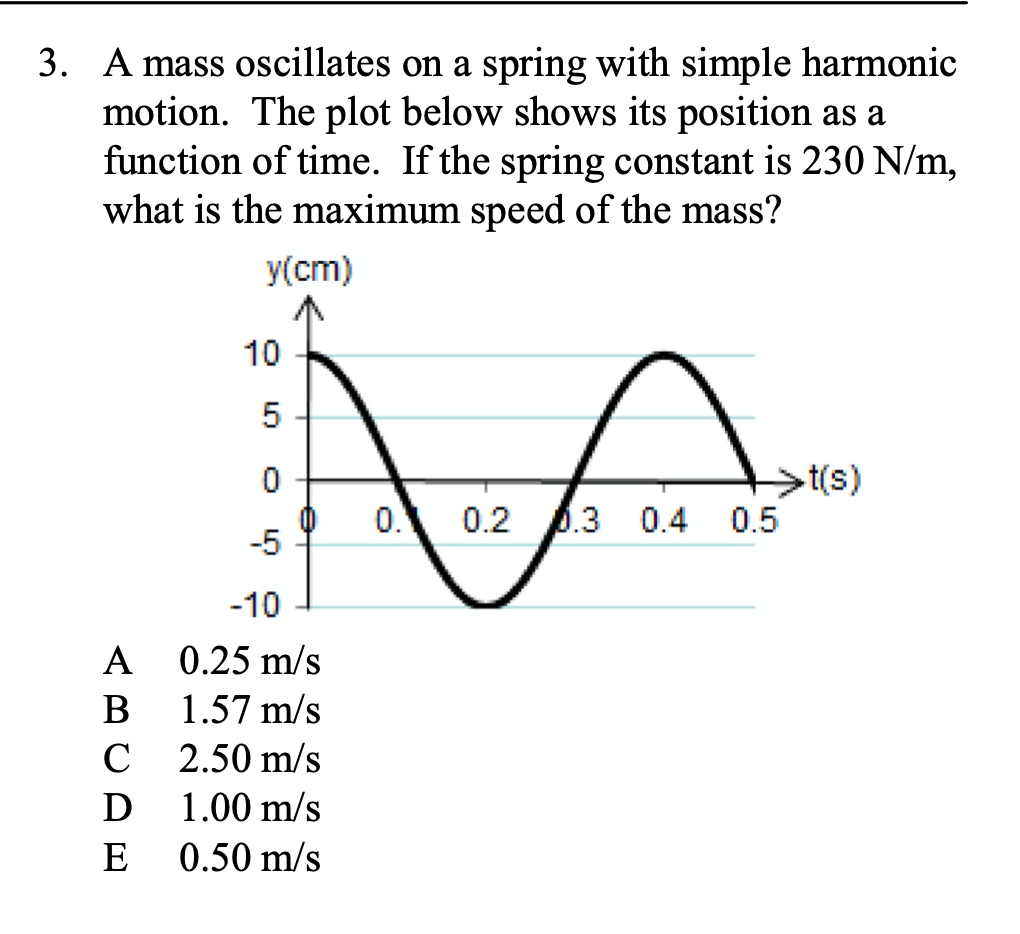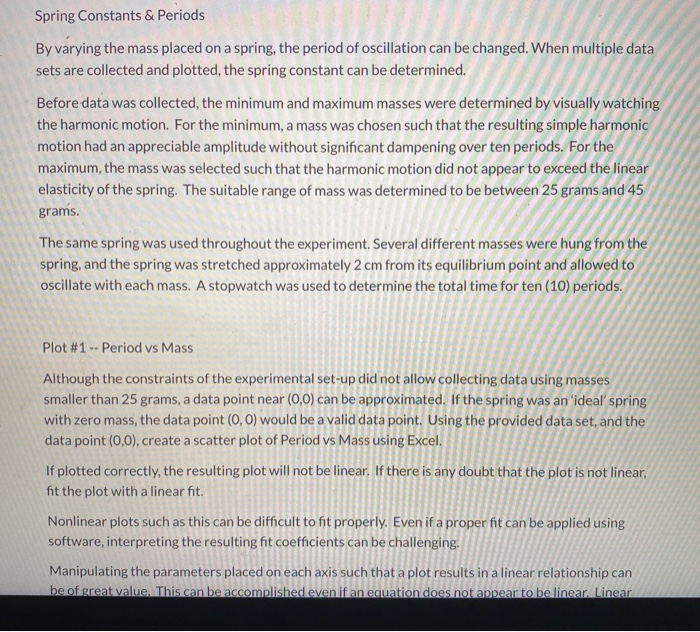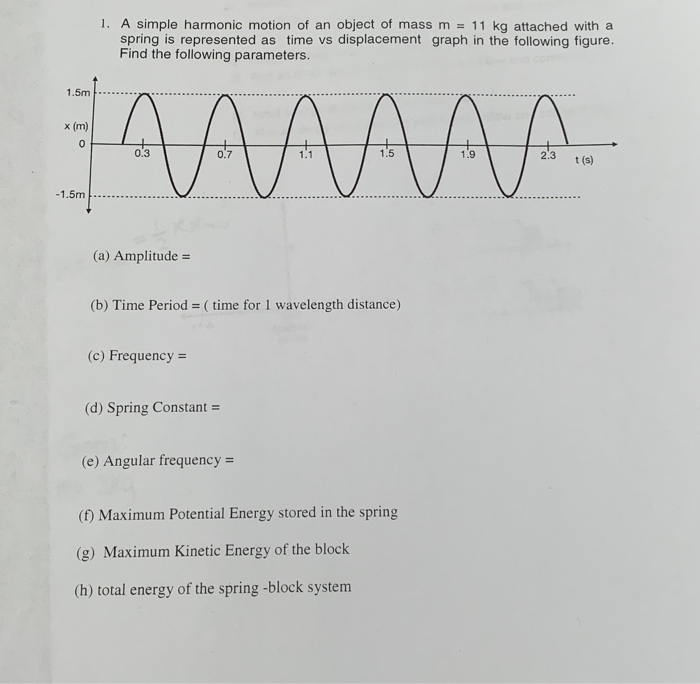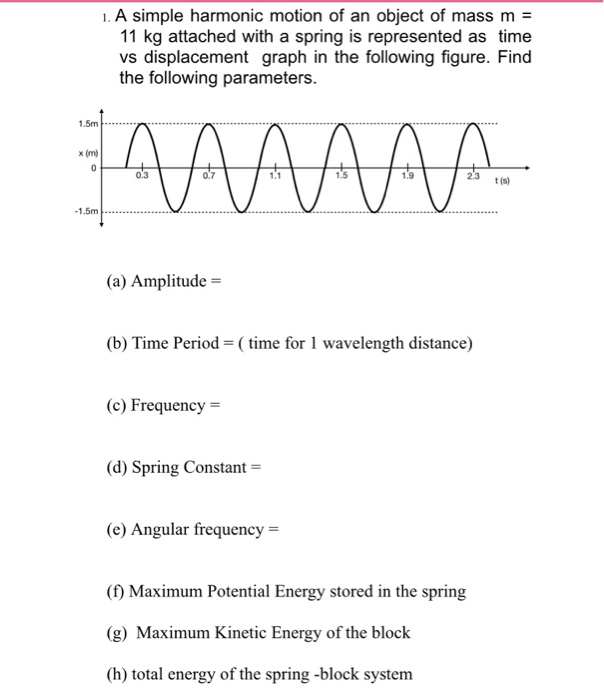Homework Help Question & Answers

# You perform the Simple Harmonic Motion experiment and create a plot of Period-Squared vs. Mass. You...

You perform the Simple Harmonic Motion experiment and create a plot of Period-Squared vs. Mass. You add a linear fit and find the following equation of the line: y=9.52x-0.067

What is the spring constant, k (in N/m or kg/s2)?

#### Homework Answers

Answer #1

The time period of a SHM is

T = 2*pi * sqrt(m/k)

where m is the mass and k is the spring constant.

So, T^2 /m = 4*pi^2 /k

From the equation of the line, we can deduce that T^2 /m is nothing but the slope of the line.

So, T^2 /m = 9.52

So, 9.52 = 4*pi^2 /k

k = 4.15 N/m

Know the answer?
Your Answer:

#### Post as a guest

Your Name:

What's your source?

#### Earn Coin

Coins can be redeemed for fabulous gifts.

Not the answer you're looking for? Ask your own homework help question. Our experts will answer your question WITHIN MINUTES for Free.
Similar Homework Help Questions
• ### You perform the Hooke's Law experiment and create a plot of Displacement vs. Force. You add...

You perform the Hooke's Law experiment and create a plot of Displacement vs. Force. You add a linear fit and find the following equation for the line: y=0.192 x - 0.011 What is the spring constant, k (in N/m or kg/s2)?

• ### A 0.50 kg mass oscillates in simple harmonic motion on a spring with a spring constant...

A 0.50 kg mass oscillates in simple harmonic motion on a spring with a spring constant of 210 N/m . Part A What is the period of the oscillation? Part B What is the frequency of the oscillation?

• ### 1) A 12.3 kg particle is undergoing simple harmonic motion with an amplitude of 1.86 mm....

1) A 12.3 kg particle is undergoing simple harmonic motion with an amplitude of 1.86 mm. The maximum acceleration experienced by the particle is 7.93 km/s2. (a) Find the period of the motion. (b) What is the maximum speed of the particle? (c) Calculate the total mechanical energy of this simple harmonic oscillator. 2) The orbit of the Moon around the Earth as projected along a diameter can be viewed as simple harmonic motion. Calculate the effective force constant k...

• ### 3. A mass oscillates on a spring with simple harmonic motion. The plot below shows its...3. A mass oscillates on a spring with simple harmonic motion. The plot below shows its position as a function of time. If the spring constant is 230 N/m, what is the maximum speed of the mass? y(cm) 10h 5 0+ 0. 0.2 1.3 0.4 >t(s) 0.5 -101 A B C D E 0.25 m/s 1.57 m/s 2.50 m/s 1.00 m/s 0.50 m/s

• ### Spring Constants & Periods By varying the mass placed on a spring, the period of oscillation...Spring Constants & Periods By varying the mass placed on a spring, the period of oscillation can be changed. When multiple data sets are collected and plotted, the spring constant can be determined. Before data was collected, the minimum and maximum masses were determined by visually watching the harmonic motion. For the minimum, a mass was chosen such that the resulting simple harmonic motion had an appreciable amplitude without significant dampening over ten periods. For the maximum, the mass was...

• ### A spring-mass system is in simple harmonic motion. How do the period, maximum speed, frequency, and...

A spring-mass system is in simple harmonic motion. How do the period, maximum speed, frequency, and total mechanical energy of the oscillator change after each of the following alterations (up, down or no change): a) Spring constant (k) is increased? b) Amplitude id increased? c) Mass is decreased?

• ### A steel ball attached to a spring moves in simple harmonic motion. The amplitude of the ball's motion is 10.0 cm, and th...

A steel ball attached to a spring moves in simple harmonic motion. The amplitude of the ball's motion is 10.0 cm, and the spring constant is 6.00 N/m. When the ball is halfway between its equilibrium position and its maximum displacement from equilibrium, its speed is 19.7 cm/s. (a) What is the mass of the ball (in kg)? kg (b) What is the period of oscillation (in s)? s (c) What is the maximum acceleration of the ball? (Enter the...

• ### 1. A simple harmonic motion of an object of mass m = 11 kg attached with a spring is represented as time vs displac...1. A simple harmonic motion of an object of mass m = 11 kg attached with a spring is represented as time vs displacement graph in the following figure. Find the following parameters. 1.5m - АААААА 0.3 23 23 tis) -1.5m (a) Amplitude = (b) Time Period = (time for 1 wavelength distance) (c) Frequency = (d) Spring Constant = (e) Angular frequency = (f) Maximum Potential Energy stored in the spring (g) Maximum Kinetic Energy of the block (h)...

• ### 1. A simple harmonic motion of an object of mass m = 11 kg attached with...1. A simple harmonic motion of an object of mass m = 11 kg attached with a spring is represented as time vs displacement graph in the following figure. Find the following parameters. (a) Amplitude = (b) Time Period = ( time for 1 wavelength distance) (c) Frequency = (d) Spring Constant = (e) Angular frequency = (f) Maximum Potential Energy stored in the spring (g) Maximum Kinetic Energy of the block (h) total energy of the spring -block system

Free Homework App

Scan Your Homework
to Get Instant Free Answers
Need Online Homework Help?

Get Answers For Free
Most questions answered within 3 hours.